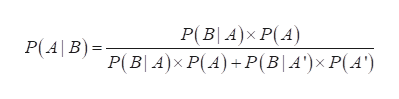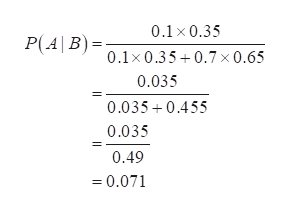The probability of event A, given that event B has occurred, can be found using Bayes’s Theorem.Use Bayes’s Theorem to find P(A | B) using the probabilities shown below P(A)=0.35, P(A’)=0.65, P(B | A)=0.1, and P(B | A’)=0.7The probability of event A, given that event B has occurred, is(Round to the nearest thousandth as needed)

Question

The probability of event A, given that event B has occurred, can be found using Bayes’s Theorem.

Use Bayes’s Theorem to find P(A | B) using the probabilities shown below P(A)=0.35, P(A’)=0.65, P(B | A)=0.1, and P(B | A’)=0.7

The probability of event A, given that event B has occurred, is

(Round to the nearest thousandth as needed)

Step 1

Given that, P(A) = 0.35 , P(A') = 0.65, P(B | A) = 0.1 and

P(B | A') = 0.7

Step 2

Baye’s theorem:help_outlineImage TranscriptioncloseP(BA)x P(A) P(4\B)= P(B| 4)x P(A) +P(B| A')x P(A') fullscreen
Step 3

Calculation:

...help_outlineImage Transcriptionclose0.1 x 0.35 P(AB)= 0.1x0.35+0.7 x0.65 0.035 0.035 0.455 0.035 0.49 =0.071 fullscreen

Want to see the full answer?

See Solution

Want to see this answer and more?

Our solutions are written by experts, many with advanced degrees, and available 24/7

See Solution
Tagged in

Hypothesis Testing